# Java Script MCSBCS Java Script Output Java Script

• Slides: 20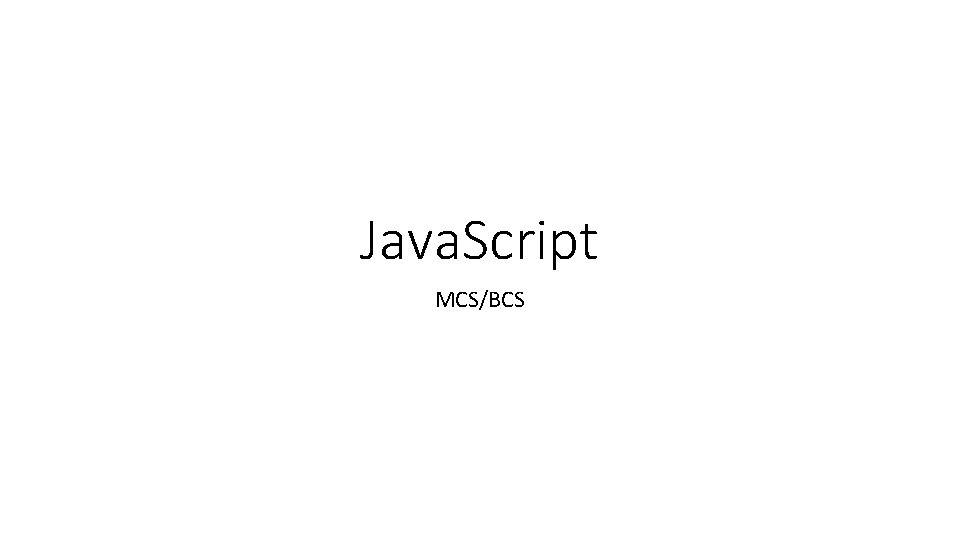Java. Script MCS/BCS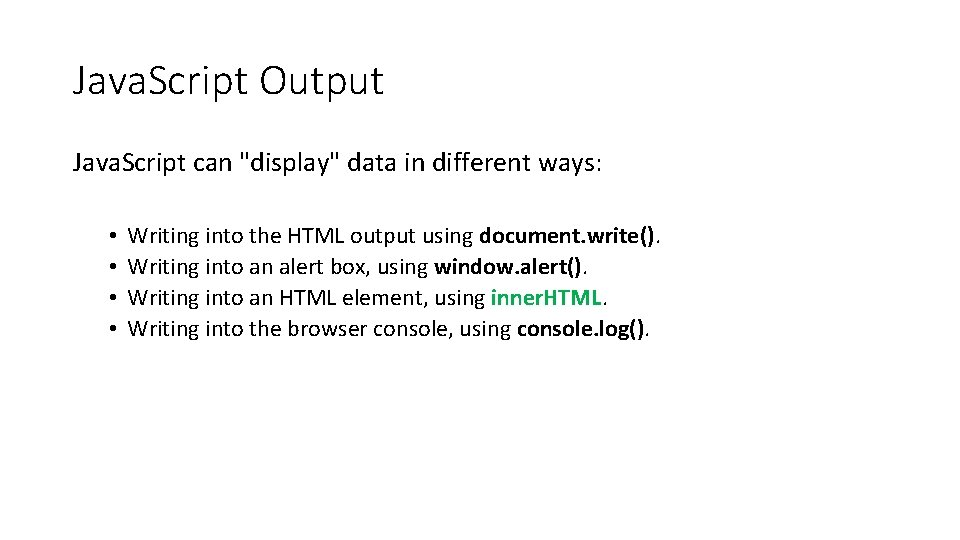Java. Script Output Java. Script can "display" data in different ways: • • Writing into the HTML output using document. write(). Writing into an alert box, using window. alert(). Writing into an HTML element, using inner. HTML. Writing into the browser console, using console. log().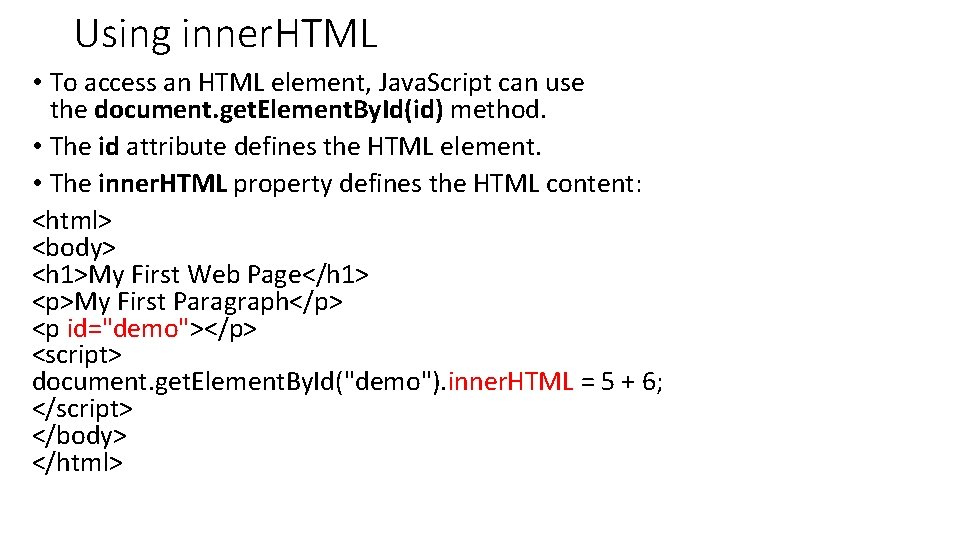Using inner. HTML • To access an HTML element, Java. Script can use the document. get. Element. By. Id(id) method. • The id attribute defines the HTML element. • The inner. HTML property defines the HTML content: <html> <body> <h 1>My First Web Page</h 1> <p>My First Paragraph</p> <p id="demo"></p> <script> document. get. Element. By. Id("demo"). inner. HTML = 5 + 6; </script> </body> </html>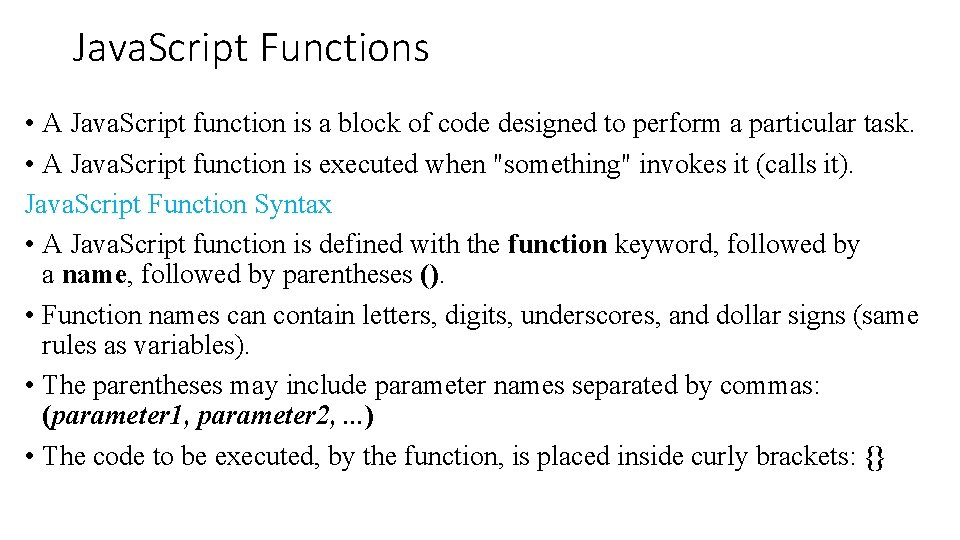Java. Script Functions • A Java. Script function is a block of code designed to perform a particular task. • A Java. Script function is executed when "something" invokes it (calls it). Java. Script Function Syntax • A Java. Script function is defined with the function keyword, followed by a name, followed by parentheses (). • Function names can contain letters, digits, underscores, and dollar signs (same rules as variables). • The parentheses may include parameter names separated by commas: (parameter 1, parameter 2, . . . ) • The code to be executed, by the function, is placed inside curly brackets: {}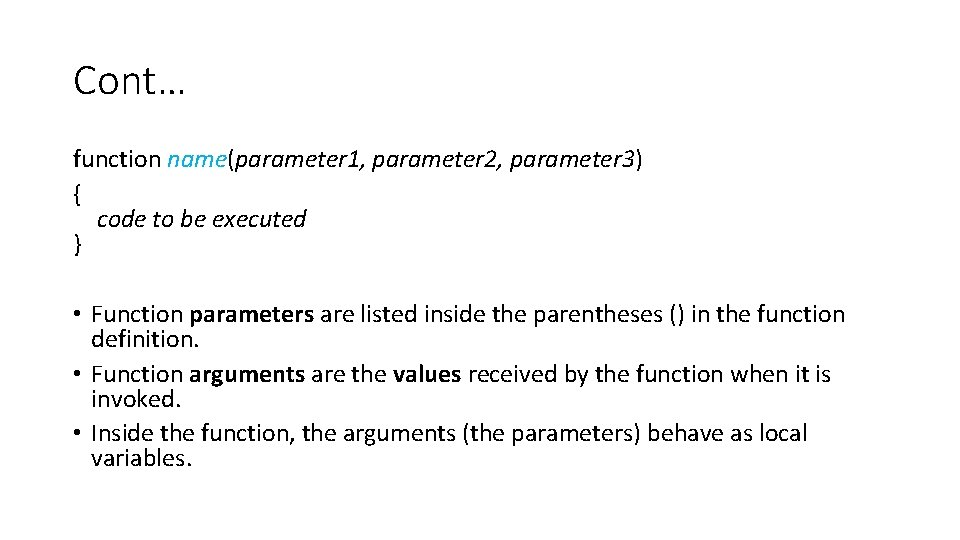Cont… function name(parameter 1, parameter 2, parameter 3) { code to be executed } • Function parameters are listed inside the parentheses () in the function definition. • Function arguments are the values received by the function when it is invoked. • Inside the function, the arguments (the parameters) behave as local variables.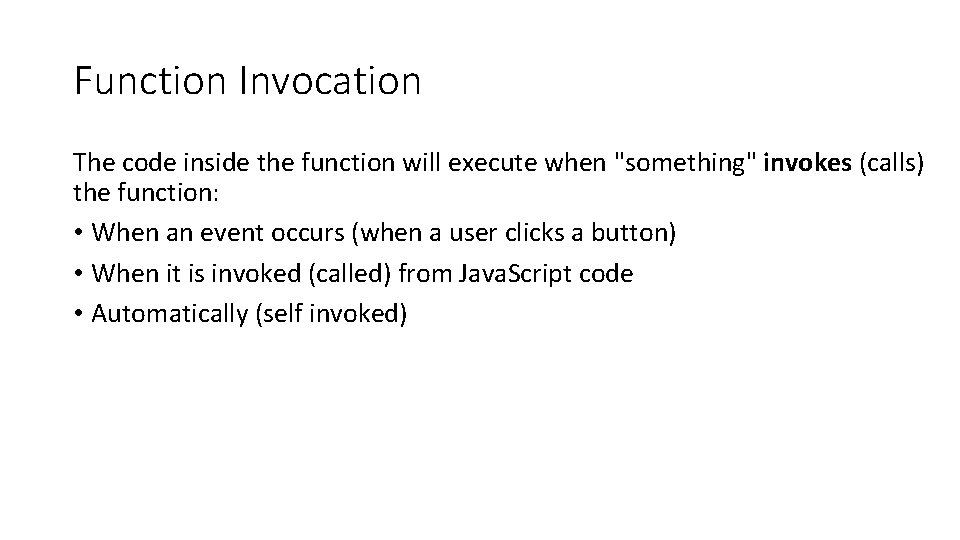Function Invocation The code inside the function will execute when "something" invokes (calls) the function: • When an event occurs (when a user clicks a button) • When it is invoked (called) from Java. Script code • Automatically (self invoked)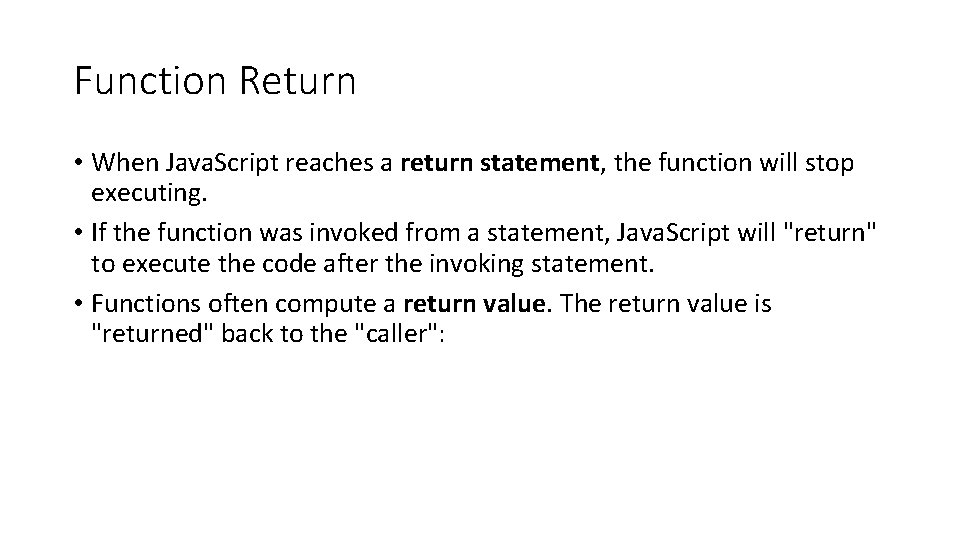Function Return • When Java. Script reaches a return statement, the function will stop executing. • If the function was invoked from a statement, Java. Script will "return" to execute the code after the invoking statement. • Functions often compute a return value. The return value is "returned" back to the "caller":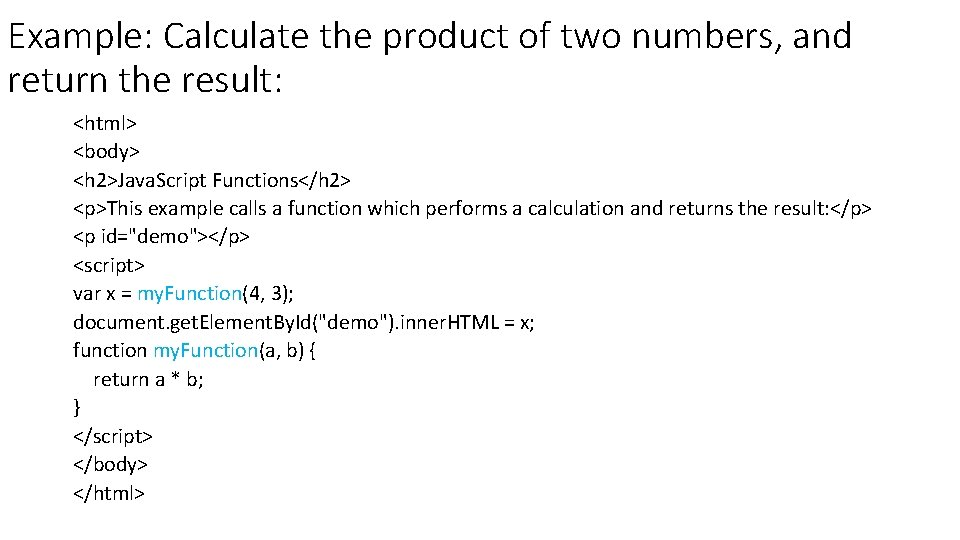Example: Calculate the product of two numbers, and return the result: <html> <body> <h 2>Java. Script Functions</h 2> <p>This example calls a function which performs a calculation and returns the result: </p> <p id="demo"></p> <script> var x = my. Function(4, 3); document. get. Element. By. Id("demo"). inner. HTML = x; function my. Function(a, b) { return a * b; } </script> </body> </html>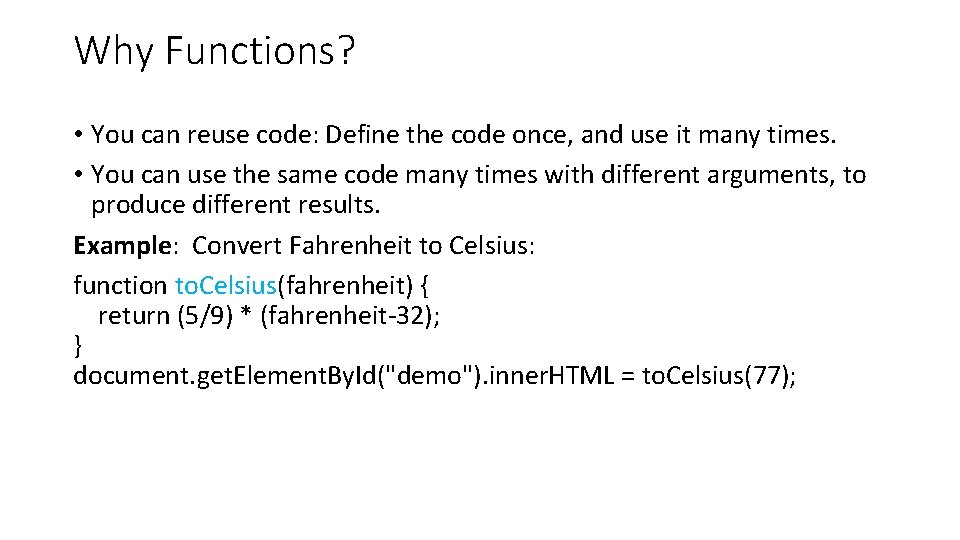Why Functions? • You can reuse code: Define the code once, and use it many times. • You can use the same code many times with different arguments, to produce different results. Example: Convert Fahrenheit to Celsius: function to. Celsius(fahrenheit) { return (5/9) * (fahrenheit-32); } document. get. Element. By. Id("demo"). inner. HTML = to. Celsius(77);Functions Used as Variable Values Functions can be used the same way as you use variables, in all types of formulas, assignments, and calculations. Example Instead of using a variable to store the return value of a function: var x = to. Celsius(77); var text = "The temperature is " + x + " Celsius"; You can use the function directly, as a variable value: var text = "The temperature is " + to. Celsius(77) + " Celsius";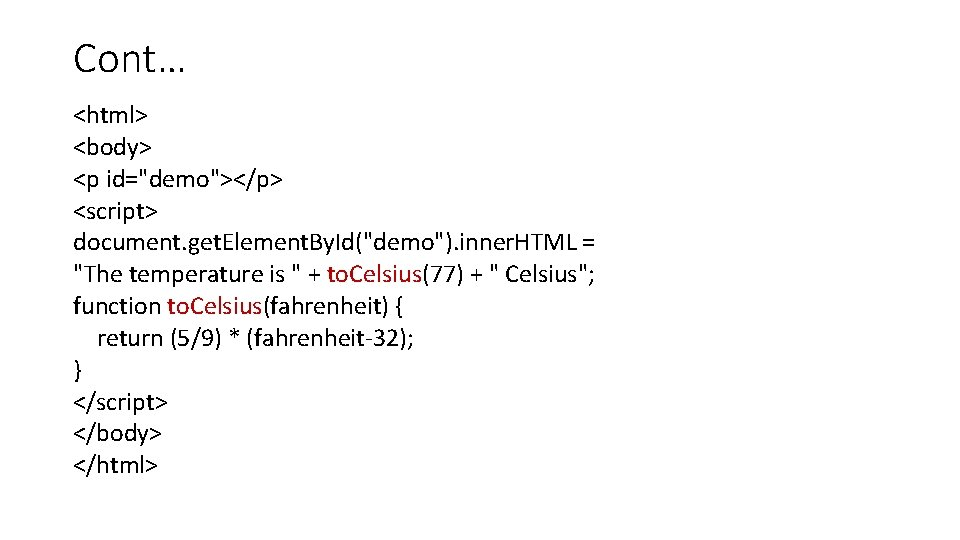Cont… <html> <body> <p id="demo"></p> <script> document. get. Element. By. Id("demo"). inner. HTML = "The temperature is " + to. Celsius(77) + " Celsius"; function to. Celsius(fahrenheit) { return (5/9) * (fahrenheit-32); } </script> </body> </html>Java. Script Objects Real Life Objects, Properties, and Methods In real life, a car is an object. A car has properties like model, weight and color, and methods like start and stop: • All cars have the same properties, but the property values differ from car to car. • All cars have the same methods, but the methods are performed at different times.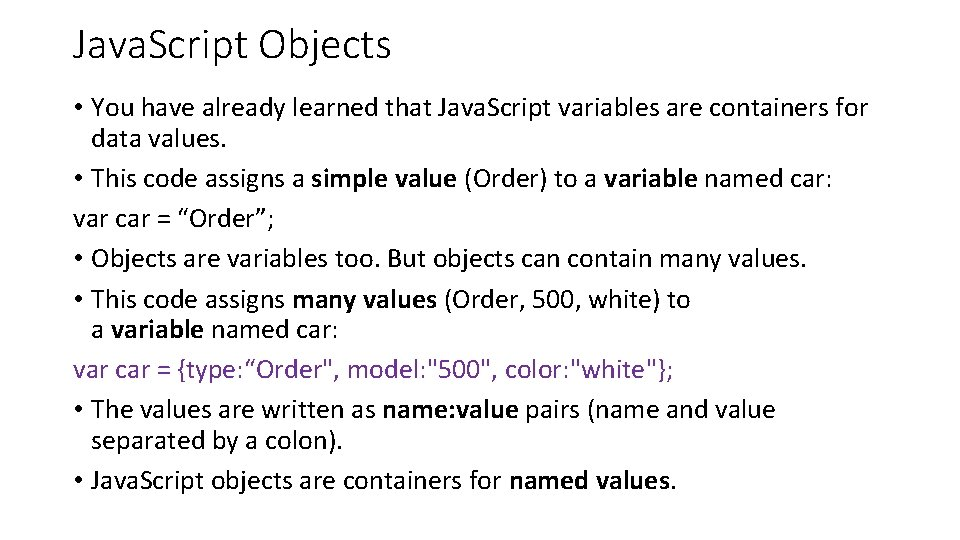Java. Script Objects • You have already learned that Java. Script variables are containers for data values. • This code assigns a simple value (Order) to a variable named car: var car = “Order”; • Objects are variables too. But objects can contain many values. • This code assigns many values (Order, 500, white) to a variable named car: var car = {type: “Order", model: "500", color: "white"}; • The values are written as name: value pairs (name and value separated by a colon). • Java. Script objects are containers for named values.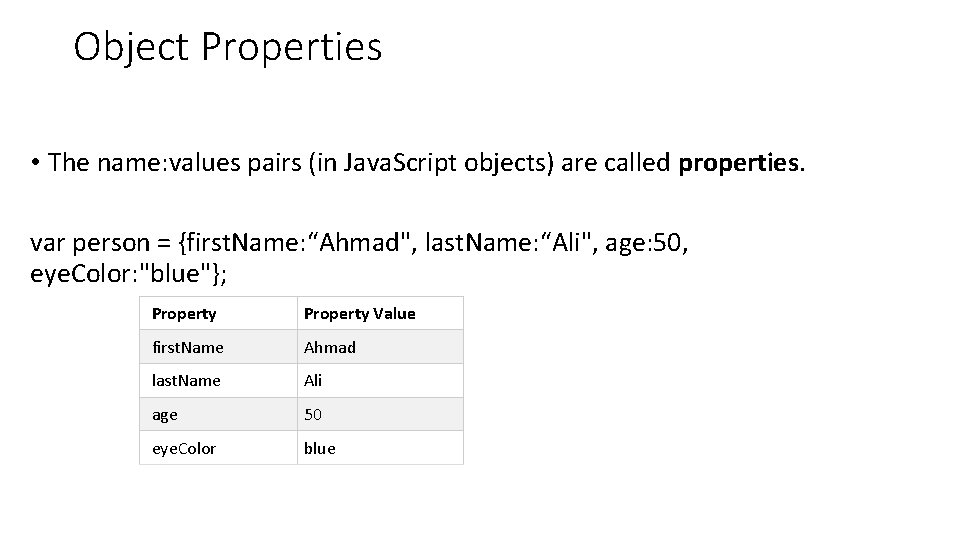Object Properties • The name: values pairs (in Java. Script objects) are called properties. var person = {first. Name: “Ahmad", last. Name: “Ali", age: 50, eye. Color: "blue"}; Property Value first. Name Ahmad last. Name Ali age 50 eye. Color blue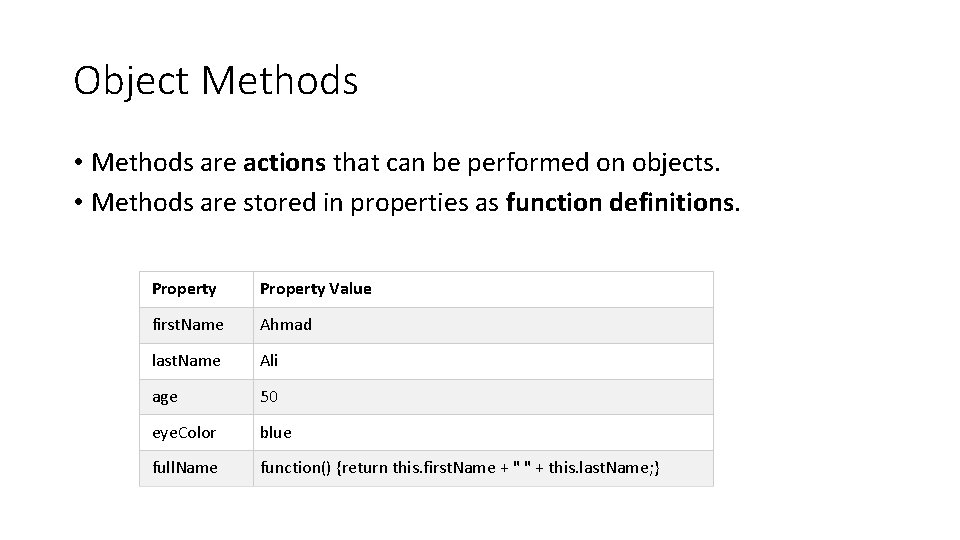Object Methods • Methods are actions that can be performed on objects. • Methods are stored in properties as function definitions. Property Value first. Name Ahmad last. Name Ali age 50 eye. Color blue full. Name function() {return this. first. Name + " " + this. last. Name; }Object Definition • You define (and create) a Java. Script object with an object literal: Example var person = {first. Name: “Ahmad", last. Name: “Ali", age: 50, eye. Color: "blue"}; OR var person = { first. Name: “Ahmad", last. Name: “Ali", age: 50, eye. Color: "blue" };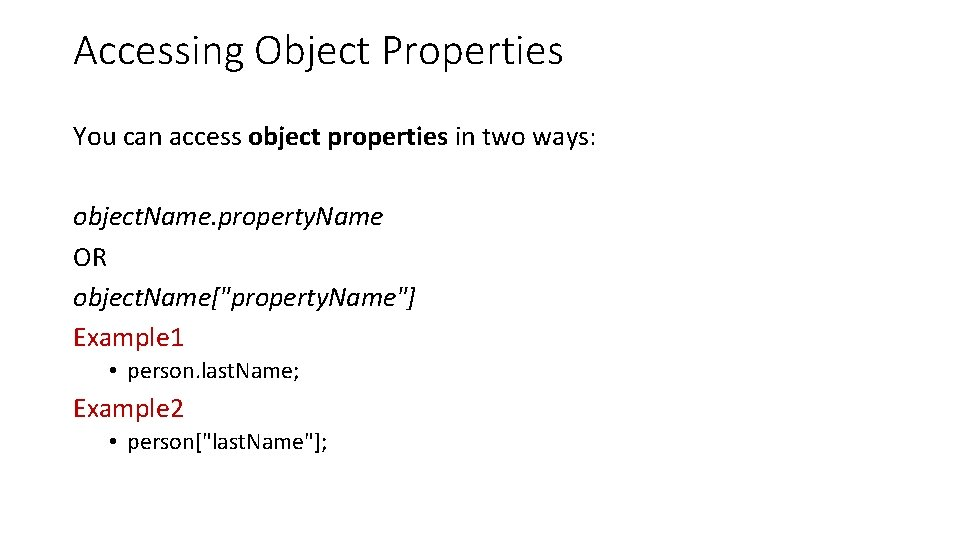Accessing Object Properties You can access object properties in two ways: object. Name. property. Name OR object. Name["property. Name"] Example 1 • person. last. Name; Example 2 • person["last. Name"];Example <html> <body> <p> There are two different ways to access an object property: </p> <p>You can use person. property or person["property"]. </p> <p id="demo"></p> <script> var person = { first. Name: “Ahamd", last. Name : “Ali", id : 5566 }; document. get. Element. By. Id("demo"). inner. HTML = person["first. Name"] + " " + person["last. Name"]; </script> </body> </html> Output: Ahmad Ali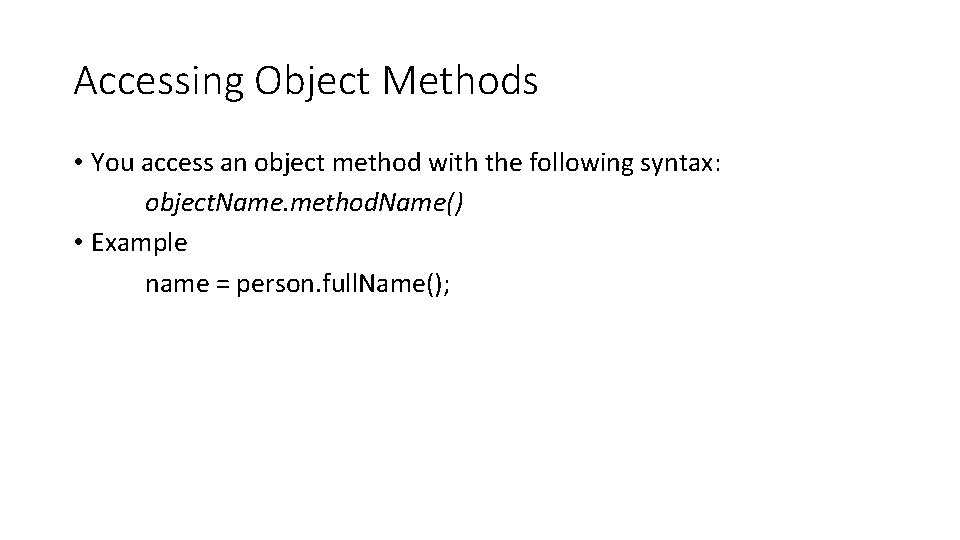Accessing Object Methods • You access an object method with the following syntax: object. Name. method. Name() • Example name = person. full. Name();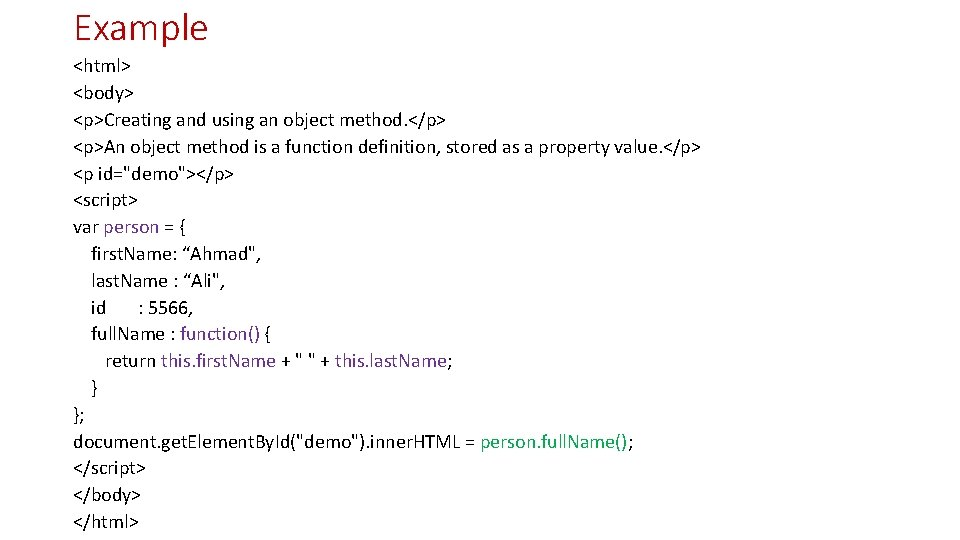Example <html> <body> <p>Creating and using an object method. </p> <p>An object method is a function definition, stored as a property value. </p> <p id="demo"></p> <script> var person = { first. Name: “Ahmad", last. Name : “Ali", id : 5566, full. Name : function() { return this. first. Name + " " + this. last. Name; } }; document. get. Element. By. Id("demo"). inner. HTML = person. full. Name(); </script> </body> </html>